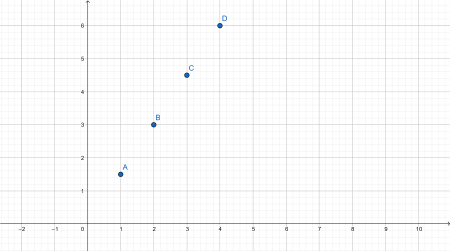## Cost Function

The total cost of purchased merchandise depends on the price and quantity purchased. The following formula could be used for the total cost:

{eq}TC = (price)\cdot (quantity)= p\cdot q {/eq}. This formula is typically used in finance or economics.

The table below shows the price, quantity, and the total cost of buying 1,2,3, or 4 bottles.

price quantity Total Cost, TC
1.50 1 1.50
1.50 2 3
1.50 3 4.50
1.50 4 6

To graph the total cost in terms of the number of bottles, we use the ordered pairs (quantity, total cost): {(1, 1.50), (2, 3). (3, 4.50), (4, 6)}. These pairs are represented by the points A,B,C, and D on the graph.Cost Function in Calculus: Formula & Examples

from

Chapter 20 / Lesson 13
112K

Learn about the cost function formula. Discover how to find cost function. Explore average cost function and marginal cost function. See examples of finding the cost.